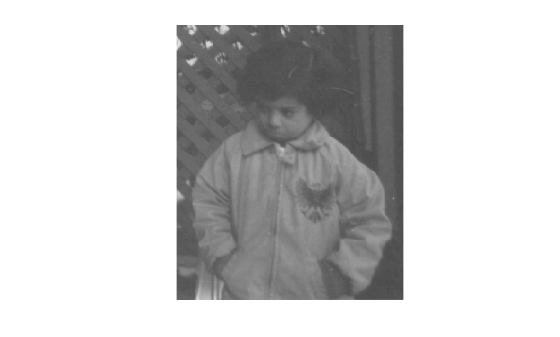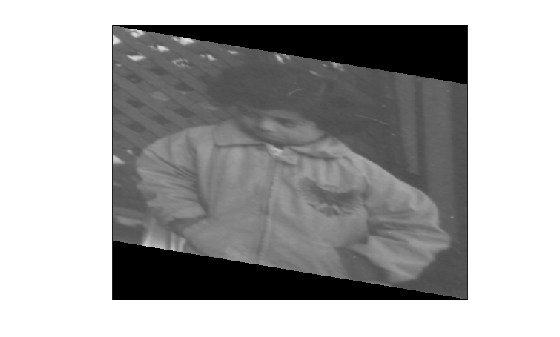# affinetform2d

2-D affine geometric transformation

Since R2022b

## Description

An `affinetform2d` object stores information about a 2-D affine geometric transformation and enables forward and inverse transformations.

## Creation

You can create an `affinetform2d` object in these ways:

• `imregtform` — Estimate a geometric transformation that maps a moving image to a fixed image using similarity optimization.

• `imregcorr` — Estimate a geometric transformation that maps a moving image to a fixed image using phase correlation.

• `fitgeotform2d` — Estimate a geometric transformation that maps pairs of control points between two images.

• `randomAffine2d` — Create a randomized 2-D affine transformation.

• The `affinetform2d` function described here.

### Syntax

``tform = affinetform2d``
``tform = affinetform2d(A)``
``tform = affinetform2d(tformIn)``

### Description

````tform = affinetform2d` creates an `affinetform2d` object that performs an identity transformation.```

example

````tform = affinetform2d(A)` creates an `affinetform2d` object and sets the property `A` as the specified 2-D affine transformation matrix.```
````tform = affinetform2d(tformIn)` creates an `affinetform2d` object from another geometric transformation object, `tformIn`, that represents a valid 2-D affine geometric transformation.```

### Input Arguments

expand all

Affine 2-D geometric transformation, specified as an `affinetform2d` object, `rigidtform2d` object, `simtform2d` object, `transltform2d` object, or `projtform2d` object.

## Properties

expand all

Forward 2-D affine transformation, specified as a 3-by-3 numeric matrix. When you create the object, you can also specify `A` as a 2-by-3 numeric matrix. In this case, the object concatenates the row vector `[0 0 1]` to the end of the matrix, forming a 3-by-3 matrix. The default value of `A` is the identity matrix.

The matrix `A` transforms the point (u, v) in the input coordinate space to the point (x, y) in the output coordinate space using the convention:

`$\left[\begin{array}{c}x\\ y\\ 1\end{array}\right]=Α×\left[\begin{array}{c}u\\ v\\ 1\end{array}\right]$`

For an affine transformation, `A` has the form:

`$Α=\left[\begin{array}{ccc}a& b& c\\ d& e& f\\ 0& 0& 1\end{array}\right]$`

Data Types: `double` | `single`

Dimensionality of the geometric transformation for both input and output points, specified as the value `2`.

## Object Functions

 `invert` Invert geometric transformation `outputLimits` Find output spatial limits given input spatial limits `transformPointsForward` Apply forward geometric transformation `transformPointsInverse` Apply inverse geometric transformation

## Examples

collapse all

Define a 3-by-3 geometric transformation matrix. This example specifies a matrix for an affine transformation consisting of vertical shear and horizontal stretch.

`A = [2 0 0; 0.33 1 0; 0 0 1];`

Create an `affinetform2d` object from the transformation matrix.

`tform = affinetform2d(A)`
```tform = affinetform2d with properties: Dimensionality: 2 A: [3x3 double] ```

```I = imread("pout.tif"); imshow(I)```Apply the geometric transformation to the image, and display the result.

```J = imwarp(I,tform); imshow(J)```## Version History

Introduced in R2022b

expand all